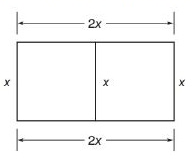Chapter 8.2, Problem 34EElementary Geometry For College St...

7th Edition
Alexander + 2 others
ISBN: 9781337614085

Solutions

Chapter
SectionElementary Geometry For College St...

7th Edition
Alexander + 2 others
ISBN: 9781337614085
Textbook Problem

The farmer in Exercise 33 has decided to take the fencing purchased and use it to enclose the subdivided plots shown.a) What are the overall dimensions of the rectangular enclosure shown?b) What is the total area of the enclosure shown?To determine

(a)

To find:

The overall dimensions of the rectangular enclosure.

Explanation

It is given that the farmer has decided to take the fencing purchased (770 ft as per previous problem ) and use it to enclose the subdivided plots as shown below.

So, the total amount of enclosure is 2x+2x+x+x+x=7x.

The overall dimensions of the rectangular enclosure provided

To determine

(b)

To find:

The total area of the enclosure.

Still sussing out bartleby?

Check out a sample textbook solution.

See a sample solution

The Solution to Your Study Problems

Bartleby provides explanations to thousands of textbook problems written by our experts, many with advanced degrees!

Get Started

In Exercises 19-24, find the functions f + g, f g, fg, and fg. 22. f(x) =1x2+1; g(x) = 1x21

Applied Calculus for the Managerial, Life, and Social Sciences: A Brief Approach

Verify by differentiation that the formula is correct. tan2xdx=tanxx+C

Single Variable Calculus: Early Transcendentals, Volume I

What are the two requirements for a random sample?

Essentials of Statistics for The Behavioral Sciences (MindTap Course List)

In problems 63-73, factor each expression completely. 67.

Mathematical Applications for the Management, Life, and Social Sciences

The directrix of the conic given by r=62+10sin is: a) x=53 b) x=35 c) y=53 d) y=35

Study Guide for Stewart's Single Variable Calculus: Early Transcendentals, 8th

The area of the parallelogram at the right is: 26

Study Guide for Stewart's Multivariable Calculus, 8th